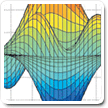Cody

Problem 937. Rubik's Mini Cube: Solve Randomized Cube in 11 Moves or Less; Score is by Time (msec)

The Challenge is to Near or Optimally Solve a thoroughly scrambled Mini-Rubik's Cube(2x2x2).

Any Mini-Cube can be solved in 11 or fewer Face Moves. There are only 3,674,160 unique cube positions, with only 2344 requiring the fulll 11 moves. Cube moves do not count.

The Performance metric is cumulative Time to Solve 500 cubes (msec).

A standard Mini-Rubik's Cube is shown in 3-D and also unfolded to identify the specific Tile-face/Vector numbering.

The faces are White-Up / Red-Left / Blue-Front / Orange-Right / Yellow-Down / Green-Back (ULFRDB)

Moves are denoted as F for clockwise rotation of the Front face. F'(or FP) is CCW and F2 is F twice. The provided function r_new=rubick_rot_mini(mov,r) implements moves 1-18: UFDLBRU'F'D'L'B'R'U2F2D2L2B2R2.Input: (rubik)
rubik: row vector of size 24
(The cube started [L=0,U=1,F=2,D=3,B=4,R=5] and then receives forty face moves.
Output: move_vec (Numeric of moves to solve)
move_vec:values 1:27 for UFDLBR U'F'D'L'B'R' U2F2D2L2B2R2 XYZX'Y'Z'X2Y2Z2
• Example:
• If the cube was randomized by [1 9] UD', one solution is [3 7] which are the complements in reverse order.
• Scoring is Time in msec to solve 500 cubes
• Cube Moves X, Y, and Z do not constitute a move but are needed in the vector
• A string to numeric value function is provided in the template
• Verifications will be by executing your move vector against the provided Rubik and counting number of face moves.

The function rubik_rot_mini(mov,r) is provided in the template. Other functions are provided to re-orient the cube in Cody Challenge 931, Rubik's Mini-Cube.

The Challenge Challenge 931 Rubik's Mini-Cube contains a 3D Mini-Cube Viewer for program development.

(Note: Mini-Cube can use the full cube moves and ignore edge effects)

Coming Soon: Matlab Tetris

Solution Stats

0.0% Correct | 100.0% Incorrect
Last solution submitted on Nov 08, 2018